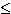User Name Remember Me? Password

 Electricity and Magnetism Electricity and Magnetism Physics Help ForumFeb 24th 2010, 06:18 PM #1 Junior Member   Join Date: Feb 2010 Posts: 2 Total charge of sphere with varying charge density? "A nonconducting solid sphere of radius R has a volume charge density that is proportional to the distance from the center. That is, ρ = Ar for rR, where A is a constant. (Use pi for π, epsilon_0 for ε0, A for A, r for r, and R for R, as necessary.) (a) Find the total charge on the sphere." When I read this I thought of there being an infinite number of infinitely small, hollow spheres ("dr") each with its own charge density. I tried taking the integral of A*r from 0 to R and I end up with A*R^2/2. I take this number to be p, and then multiplied it by V (=4/3*pi*R^3) and I get [4/3*pi*R^3*A*R/2] which then simplifies to [2/3*pi*R^4*A] which the website we enter our answers into says is incorrect. I know how simple this is if the charge density was uniform, but I don't understand how I am supposed to take into account the variations in the charge density... any help would be much appreciated!Feb 24th 2010, 06:59 PM   #2
Senior Member

Join Date: Apr 2008
Posts: 815
 Originally Posted by dspaulding09"A nonconducting solid sphere of radius R has a volume charge density that is proportional to the distance from the center. That is, ρ = Ar for rR, where A is a constant. (Use pi for π, epsilon_0 for ε0, A for A, r for r, and R for R, as necessary.) (a) Find the total charge on the sphere." When I read this I thought of there being an infinite number of infinitely small, hollow spheres ("dr") each with its own charge density. I tried taking the integral of A*r from 0 to R and I end up with A*R^2/2. I take this number to be p, and then multiplied it by V (=4/3*pi*R^3) and I get [4/3*pi*R^3*A*R/2] which then simplifies to [2/3*pi*R^4*A] which the website we enter our answers into says is incorrect. I know how simple this is if the charge density was uniform, but I don't understand how I am supposed to take into account the variations in the charge density... any help would be much appreciated!
Welcome to PHF!
I'm not really sure about the integral you've made.
Have you taken calculus 3 yet? In other words, do you know how to deal with triple integrals?
You can't multiply this "p" by the volume. If p was the charge density of the sphere it would be ok as long as the charge density is constant. Since it is not constant, you'll have to set up a triple integral. More precisely, set up the triple integral in spherical coordinates and integrate the volume charge density. Don't forget the dV element while integrating.
If you have any difficulty on doing this, I'm willing to assist you with Latex.
Good luck.
__________________
Isaac
If the problem is too hard just let the Universe solve it.Tags charge, density, sphere, total, varyingThread ToolsShow Printable VersionEmail this Page Display ModesLinear ModeSwitch to Hybrid ModeSwitch to Threaded ModeSimilar Physics Forum Discussions Thread Thread Starter Forum Replies Last Post Tutan Electricity and Magnetism 2 Dec 1st 2015 08:40 AM jjppof Electricity and Magnetism 1 May 2nd 2013 09:35 AM chevy900ss Electricity and Magnetism 12 Mar 17th 2010 07:09 PM berestore Electricity and Magnetism 1 Mar 2nd 2010 06:57 PM reverseswing Advanced Electricity and Magnetism 0 Jun 19th 2009 02:02 AM